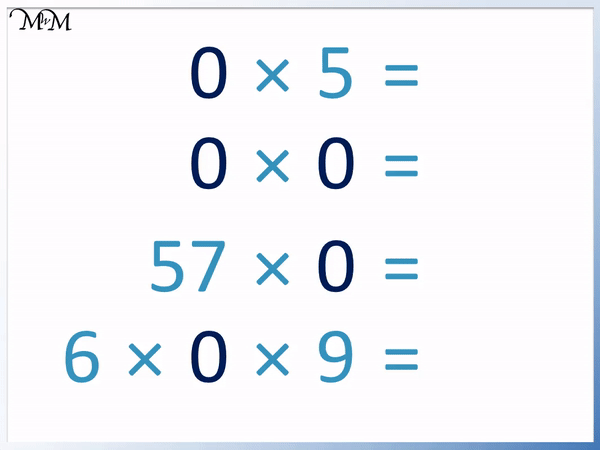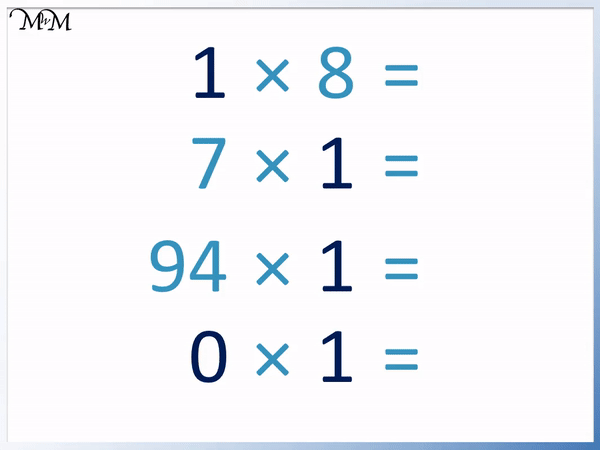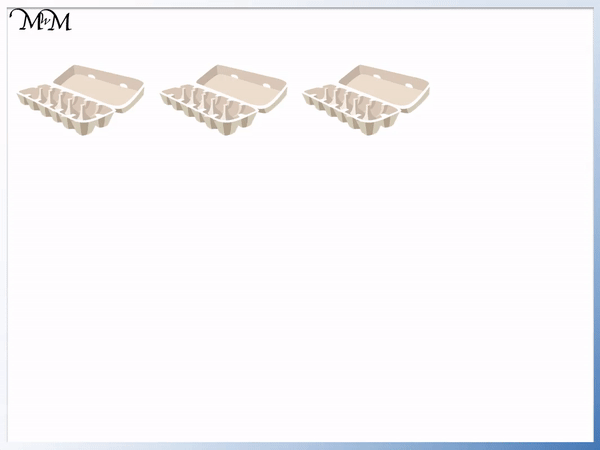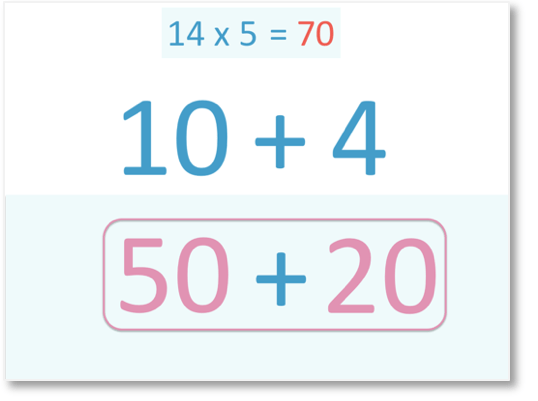# Multiplying by 0 and 1

Dividing Fractions#### Multiplying by Zero• Here are 4 empty egg cartons, each with zero eggs in.
• We have 4 lots of zero eggs.
• There are still zero eggs in total.
• 4 lots of zero is zero.
• 4 × 0 = 0.
• Any number multiplied by zero always equals zero.
• It does not matter how many numbers there are or how large they are, if we have a multiplication by 0, the answer will always be zero.
• Even if zero was written first, the answer is still zero.
• For example, 0 × 4 = 0.

#### Multiplying by One• We have 3 cartons of eggs, each containing one egg.
• We have 3 lots of 1, which is 3 eggs in total.
• We write this as 3 × 1 = 3.
• Multiplying by 1 did not make 3 any larger, it just remained the same.
• One multiplied by a given number equals the given number.
• The number multiplied by 1 is 3 and so, 3 is the answer.
• Even written the other way around, we have 1 × 3 = 3.

When we multiply by zero the answer is always zero.

When we multiply a number by one it remains the same size.#### Examples of Multiplying by 0• If there is a zero in a multiplication then the answer to a multiplication will always by zero.
• It does not matter if the zero comes first, such as in 0 × 5 = 0.
• 0 × 0 = 0 because we have zero lots of nothing.
• Even large numbers equal zero when multiplied by zero, such as 57 × 0 = 0.
• It does not matter how many numbers there are. If there is only multiplication happening and there is a zero, then the answer is zero.
• 6 × 0 × 9 = 0, we don’t need to do any calculations in this example.

#### Examples of Multiplying by 1• If we multiply by 1, then the other number stays the same.
• It does not matter if the one comes first, such as in 1 × 8 = 8.
• It does not matter if the one comes second, such as in 7 × 1 = 7.
• Even large numbers remain the same size when multiplied by one, such as 94 × 1 = 94.
• Even zero remains as zero when we multiply it by 1, such as in 0 × 1 = 0#### Multiplying by 0 and 1 Interactive Question Generator

Multiplying by 0 and 1: Interactive Questions

# How to Multiply by Zero and One

## How to Multiply by 0

The rule for multiplying any number by 0 is that we always get a result of 0. Any number times zero equals zero.

It does not matter where the zero appears in the multiplication. If the only operation is multiplication, then the answer will be zero if we multiply by 0.

To understand why this is the case, we will look at some examples of multiplication.

Here are three boxes of eggs. Each box has ten eggs. We have 3 lots of 10.

We write ‘lots of’ as a multiplication sign.

3 lots of 10 is written as 3 × 10.3 × 10 = 30 and so, there are 30 eggs in total.

If we remove a box, we now have 2 lots of 10 eggs, written as 2 × 10.2 × 10 = 20, we have 20 eggs in total.

If we remove another box, we have one lot of ten eggs, written as 1 × 10.If we remove our final box, there are no eggs left.

The number that is used to represent nothing is zero. We write zero as 0.We have no more boxes, so we have no lots of 10.

We write this as 0 × 10, which is pronounced zero times ten.

There are no eggs in total so 0 × 10 = 0.We say that zero lots of any number always equals zero.

It does not matter what the number is, if it is multiplied by zero, then the answer is zero.

In this example we have 3 empty boxes.

Each box contains zero eggs so in three boxes we say we have 3 lots of 0.3 × 0 = 0 because there are no eggs in total.

Even if we add another empty box, we are not adding any eggs. We still have zero in total.

We have 4 lots of zero, written as 4 × 0.4 × 0 = 0

We say that any number multiplied by zero always equals zero.

‘× 0’ means ‘lots of nothing’. It does not matter how much of nothing you have, it is still nothing.

Here are some examples of multiplying by zero.

Remember that it does not matter what the other numbers are, as long as we are multiplying by zero, then the answer is zero.

We can see that the simple example of 0 × 5 = 0 because no lots of 5 is nothing.0 × 0 = 0 because this means no lots of nothing. It total we have nothing.

57 × 0 = 0. It does not matter how large the other number is, 57 lots of nothing is still nothing. This is like having 57 empty boxes of eggs. There are still no eggs in any of them.

Even if there are many numbers multiplied together, the answer is still zero if we are multiplying by zero.

6 × 0 × 9 = 0, which is like 9 people who each have 6 empty boxes of eggs. There are still no eggs in total because all of the boxes are empty.

## How to Multiply by 1

The rule for multiplying any number by 1 is that the number remains the same size. When multiplying a given number by one, the answer is simply the given number.

To understand why this rule works we will look at some examples of multiplication.

Here is a single box of 10 eggs. It is just one box so we say we have one lot of ten.

We write one lot of ten as 1 × 10.1 × 10 = 10 because there are 10 eggs in total.

Here is a box of 6 eggs.

Again we have one lot of 6, which we write as 1 × 6.1 × 6 = 6.

We can see in both of these cases, the answer shown after the equals sign is simply the other number that is multiplied by 1.

We say that a given number multiplied by one equals the given number.

We can also look at examples where 1 comes second in the multiplication calculation.

Here we have 3 boxes, which each contain 1 egg.

We have 3 lots of 1.

3 lots of 1 is written as 3 × 1.3 × 1 = 3 because there are three eggs in total.

Again the answer is simply the other number in the calculation that we multiply by 1.

We say that one multiplied by a given number equals the given number.

Here are some example questions of multiplying by 1.

1 × 8 = 8. We are multiplying by 1 and the other number is 8, so the answer is 8.

It does not matter if 1 comes second in the multiplication. For example 7 × 1 = 7. We are multiplying by 1 and so, the answer is simply the other number of 7.It does not matter if the other number is large, we do not need to do any working out, we simply write the other number as our answer.

94 × 1 = 94. The answer is simply the number we are multiplying by 1.

In the last example we have 0 × 1 = 0. Even the number ‘0’ remains the same. We are multiplying by 1 and so the answer is the number that is being multiplied by 1, which is 0.

We can also solve this last example with our rule for multiplying by 0.

Any number multiplied by 0 is 0 and here we have 0 × 1 = 0. One is being multiplied by zero and so, the answer is zero.Now try our lesson on Multiplication by Partitioning where we learn how to multiply larger numbers.error: Content is protected !!